Importance: High ✭✭✭
 Author(s): Seymour, Paul D.
 Subject: Graph Theory » Basic Graph Theory » » Minors
 Keywords: coloring complete graph minor
 Posted by: mdevos on: January 6th, 2008
Conjecture   Everyvertex graph with no independent set of sizehas a complete graph on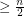vertices as a minor.

This conjecture is significant because it is an interesting unproved consequence of Hadwiger's conjecture (this implication is proved next). In fact, some experts have suggested that this problem might hold the key to finding a counterexample to Hadwiger. I (M. DeVos) have attributed this conjecture to Seymour, but I believe that it may have been independently suggested by Mader and by others. Its curious title will be explained later in this discussion.

Hadwiger's conjecture (every loopless graph with chromatic number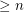hasas a minor) is one of the outstanding problems in graph theory. This conjecture has been resolved for small values of; whenit is relatively easy, for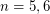it has been proven to be equivalent to the Four color theorem. The Seagull problem concerns the other extreme - when the size of the chromatic number is close to the order of the graph. Ifis anvertex graph with no independent set of size 3, then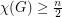since each color class has size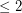. If Hadwiger's conjecture holds for, it must then have a minor which is a complete graph onvertices. This is precisely the statement of the Seagull problem.

The (essentially) best known bound for the conjecture is that everyvertex graphwith no independent set of size 3 has a complete graph onvertices as a minor. This argument is where the name of this conjecture arises. Let us call a seagull ofan induced subgraph which is a 2-edge path (such a subgraph may be drawn suggestively as a seagull). Then, for every seagulland every vertexnot in, there must be an edge betweenand one of the two endpoints of(this follows from the assumption thathas no independent set of size 3). This feature makes seagulls especially useful for constructing complete graphs as minors - as we now demonstrate. Choose a maximal collection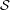of pairwise disjoint seagulls of. The graphobtained fromby deleting every vertex which appears in a seagull incannot have any two vertices at distance 2 from one another (since this would yield a seagull), somust be a disjoint union of complete graphs. Sincehas no independent set of size 3, it is in fact a disjoint union of at most two complete graphs. By deleting every vertex in the smaller complete subgraph offromand then contracting both edges in every seagull in, we obtain a complete graph minor ofwith size.

Kawarabayashi, Plummer, and Toft have improved this bound slightly by showing thatmust have a complete graph minor of size, but it looks very difficult to get much more out of this type of argument.

## Bibliography

[KPT] K. Kawarabayashi, M. Plummer, and B. Toft, Improvements of the theorem of Duchet and Meynial's theorem on Hadwiger's Conjecture, J. Combin. Theory Ser. B. 95 (2005) 152-167.

* indicates original appearance(s) of problem.